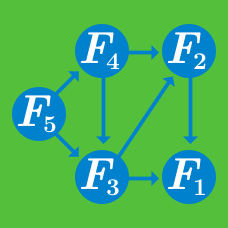Computer Science

# Backpack Problem

Suppose a thief finds himself in a vault containing several valuables. He realizes however that he brough a knapsack of capacity $M$. The vault has $n$ items, where item $i$ weighs $S_i$ pounds and is worth $v_i$ dollars. The thief must choose the items so that that he’ll make as much money as possible after selling the items. . He creates an array $optimal[i][j]$ where $optimal[i][j]$ is the maximum value obtained by using items $i ... n-1$ which weigh at most $j$ pounds.

Having never been in computer-science in college, the thief quickly scribbles four recurrence relationships for his problem. He knows only one is right. Help him find the right one.

1: $optimal[i][j] = max \{ optimal[i+1][j] , optimal[i+1][j-s_i] + v_i \ \ ( j \geq s_j ) \}$ 2: $\ \ optimal[i][j] = max \{ optimal[i+1][j+1] + v_i , optimal[i+1][j-s_i] \ \ ( j \geq s_j ) \}$ 3: $\ \ optimal[i][j] = max \{ optimal[i-1][j] , optimal[i][j+s_i] \ \ ( j \geq s_j ) \}$ 4: $\ \ optimal[i][j] = max \{ optimal[i+1][j] , optimal[i-1][j+1+s_i] \ \ ( j \geq s_j ) \}$

Give a set of eight items with a corresponding weight and value:You want to carry as many items as you can without exceeding your knapsack's weight limit. What's the maximum possible value you can get knowing that the knapsack's weight limit is $12$?

A table of eight items with their corresponding weights and values is given below.Suppose that the knapsack's weight limit is $12$, and our goal is to store the greatest possible total value inside of it. If we can only use items from the table, and we only have one of each item, what combination of items can be stored inside the bag to achieve our goal?

×